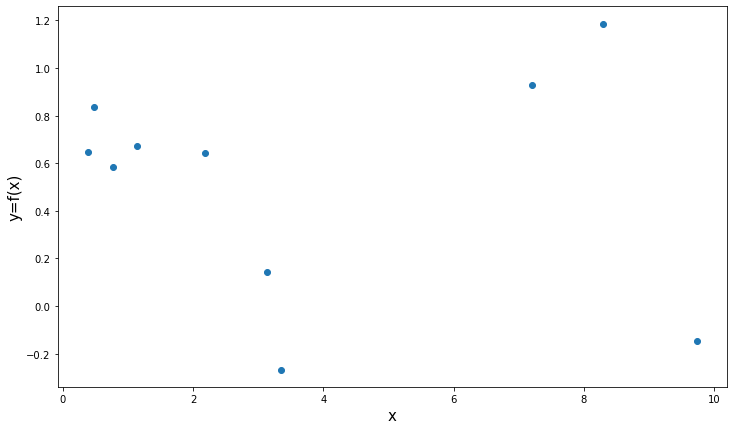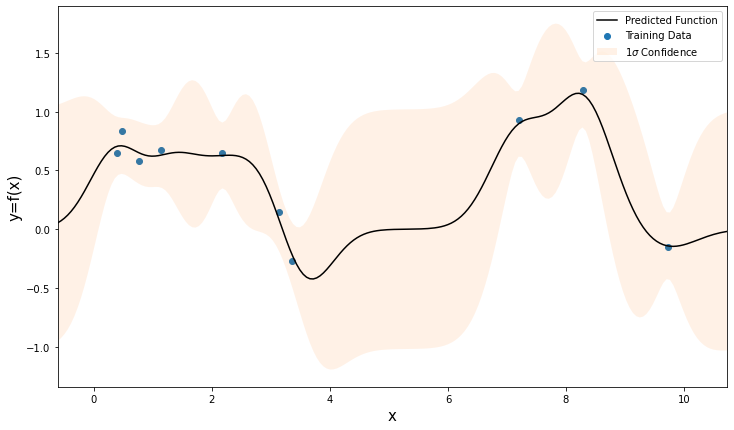In :
import numpy as np
import matplotlib.pyplot as plot

In this notebook, I present a practical example of what is actually happening when you train a Gaussian process model. I don't suggest you ever use this implementation to do actual machine learning. I have coded everything with readability in mind, at the cost of efficiency. There are also a bunch of cool linear algebra and numerical stability tricks that I have also skipped in the interest of simplicity.

The leading libraries for working with GPs (in Python) are;

• GPy - Probably the most popular
• George - Written by an astrophysicist I had beers with once, so maybe I'm biased, but this is the one I used to use as it gives the user a lot of flexibility and plays well with stochastic sampling methods
• Scikit-Learn - Used to be considered a poor implementation, not sure if that is still true

First, some notation. We have;

• $x_t$ - the inputs for our training data
• $y_t$ - the outputs for our training data
• $x_p$ - the inputs for which we want to predict the output
• $y_p$ - the (unknown) outputs that we want to predict

We generate some training data;

In :
np.random.seed(8235)

n_data = 10
measurement_uncertainty = 0.2

x_t = 10 * np.sort(np.random.rand(n_data))
y_t = np.sin(x_t)  + measurement_uncertainty * np.random.randn(len(x_t))

x_p = np.linspace(min(x_t)-1, max(x_t)+1, 200)

fig, axs = plot.subplots()
fig.set_size_inches(12,7)
axs.set_xlabel('x', size=15)
axs.set_ylabel('y=f(x)', size=15)
axs.scatter(x_t, y_t);Our model is that our outputs are jointly Gaussian distributed, i.e.,

$$P(y_p|y_t) \propto P(y_p,y_t) \propto \exp\left[-\frac{1}{2} \left(\begin{array}{c} y_t \ y_p \end{array}\right)^T\Sigma^{-1}\left(\begin{array}{c} y_t \ y_p \end{array}\right)\right]$$

Where ($y_t, y_p$) is the concatenated vector of our known and unknown outputs and $\Sigma$ is the covariance matrix between all of the outputs.

The problem is, we don't know $y_p$, so we can't compute this directly. We therefore make the assumption that we can approximate the covariance between two outputs ($y_t, y_p$) as a function of the inputs ($x_t, x_p$).

The standard choice for this function is the "squared exponential" kernel, but remember that this is just a choice!

$$\Sigma_{ij} = \langle y_i, y_j\rangle \approx \kappa(x_i, x_j) = \exp\left(-\frac{(x_i - x_j)^2 }{2l^2} \right) + \alpha\delta_{ij}$$

Where here $l$ is our free parameter, $\alpha$ is our (homoskedastic) measurement uncertainty and $\delta_{ij}$ is the Kroenecker delta function.

In :
def kernel(xi, xj, l):
"""
Remember, any positive definite, symmetric function of the inputs will do here!
"""

squared_residual = np.square(xi-xj)
covariance = np.exp(-1*squared_residual/(2*l*l))
return covariance

def kernel_matrix(xs, l):
"""
Since the matrices are symmetric, we can compute them more efficiently
"""

n = len(xs)

M = np.zeros((n, n))
for i in range(n):
for j in range(i):
M[i,j] = kernel(xs[i], xs[j], l)
M = M + M.T
for i in range(n):
M[i,i] = kernel(xs[i], xs[i], l) + measurement_uncertainty**2

return M

The first step is to figure out what the free parameters are (train the Gaussian process model).

The $\Sigma$ in the Likelihood function above contains covariances between the training outputs ($y_t$) and the predicted outputs ($y_p$). It is convenient to consider the different blocks of this matrix;

$$\Sigma = \left(\begin{array}{cc} T & C^T \\ C & P \end{array}\right)$$
• $T$ - the covariance matrix for the training outputs, made by taking pairwise covariances $\langle y_t,y_t \rangle$
• $P$ - the covariance matrix for the outputs we want to predict, made by taking pairwise covariances $\langle y_p,y_p \rangle$
• $C$ - the covariance matrix between the known, training outputs and the unknown outputs to predict $\langle y_p,y_t \rangle$

Since we're still assuming that our outputs are jointly Gaussian distributed, we use a Gaussian likelihood for this also, in order to find the most likely values for our free parameter $l$.

$$P(\alpha, l | x_t, f_t) = P(T | x_t, y_t) \propto \frac{1}{2|T|} \exp\left(-\frac{1}{2}y_t^T T^{-1} y_t \right)$$

Where the elements of $T$ can be computed using the Kernel function, which is a function of $l$.

In :
# logs are more stable, and are monotonic so work for maximisation
# since l must be positive, we pass its logarithm for optimisation later
def neg_log_likelihood(log_l):

l = np.exp(log_l)

T = kernel_matrix(x_t, l)

# multivariate Gaussian log likelihood
ll = -0.5*np.log(np.linalg.det(T)) - (0.5*np.dot(y_t,np.linalg.solve(T, y_t))) - np.log(2.*np.pi)

print(f"Log Likelihood: {ll}")

return -ll

For simplicity, I will optimise the likelihood using a scipy routine, but it's quite common to use a full Bayesian treatment here, since Gaussian likelihoods are very fast to compute.

In :
from scipy.optimize import minimize
In :
print('Optimising l:')
print()
l0 = np.log(0.5)
result = minimize(neg_log_likelihood, l0)
l_max = result.x
print()
print(f"Best l = {np.exp(l_max)}")
Optimising l:

Log Likelihood: -1.2038508653292523
Log Likelihood: -1.2038508247602695
Log Likelihood: 1.4986278795149
Log Likelihood: 1.498627896855321
Log Likelihood: -1.0046019674290614
Log Likelihood: -1.0046021266283782
Log Likelihood: 1.5969944183769633
Log Likelihood: 1.5969944224813575
Log Likelihood: 1.6017623612261378
Log Likelihood: 1.6017623604491251
Log Likelihood: 1.6019256745168962
Log Likelihood: 1.6019256745553445
Log Likelihood: 1.6019260806170736
Log Likelihood: 1.6019260806174316
Log Likelihood: 1.6019260806518476
Log Likelihood: 1.6019260806518472

Best l = 1.6259474735691932

Now that we have our optimised value for $l$, we can compute all of the other values of $\Sigma$. From this, we can plug straight in to the Gaussian process update equations (the derivation of these is long, but you can see the full derivation on some loser's blog here), which define a multivariate Gaussian distribution from which our function is drawn

$$P(y_p|y_t) \sim \mathcal{N}(CT^{-1}y_t, P-CT^{-1}C^T)$$

So that our predictions are given by the mean vector

$$\mu \approx CT^{-1}y_t$$

with uncertainty summarised by the covariance matrix

$$\Sigma \approx P-CT^{-1}C^T$$
In :
# compute the parts of the matrix

C = np.zeros((len(x_p), len(x_t)))
for i in range(len(x_p)):
for j in range(len(x_t)):
C[i, j] = kernel(x_p[i], x_t[j], l_max)

P = kernel_matrix(x_p, l_max)
T = kernel_matrix(x_t, l_max)
In :
# get our distribution over functions

mu_p = np.dot(C, np.linalg.solve(T, y_t))
cov_p = P - np.dot(C, np.dot(np.linalg.inv(T), C.T))
sig_p = np.sqrt(np.diag(cov_p))

We now have the mean and covariance of our function, so we can show our best guess, with uncertainty, of what our approximation might look like

In :
fig, axs = plot.subplots()

axs.scatter(x_t, y_t, label='Training Data')
axs.fill_between(x_p, mu_p - sig_p, mu_p + sig_p, alpha=0.1, label=r'$1\sigma$ Confidence')
axs.plot(x_p, mu_p, label='Predicted Function', color='k')
axs.set_xlim(min(x_p),max(x_p))
axs.legend()

axs.set_xlabel('x', size=15)
axs.set_ylabel('y=f(x)', size=15)

fig.set_size_inches(12,7)